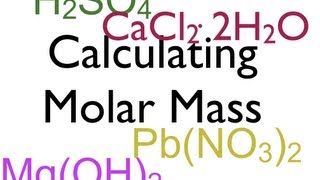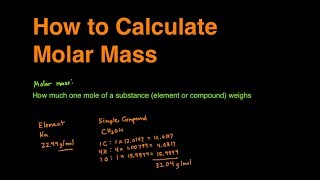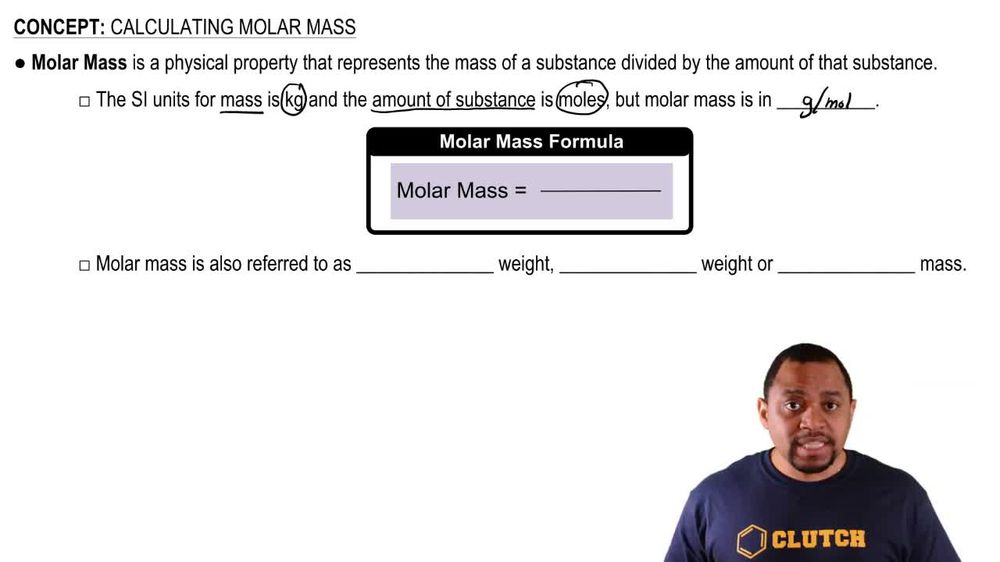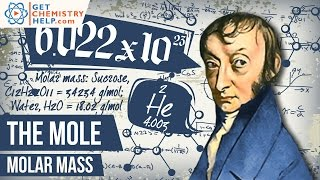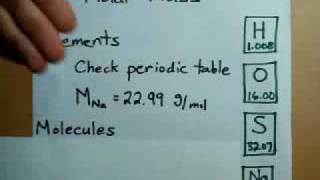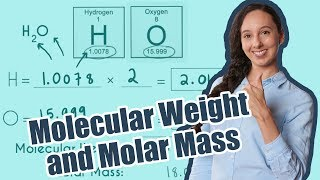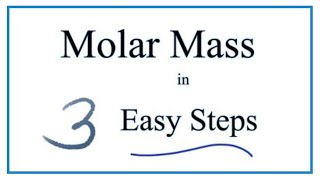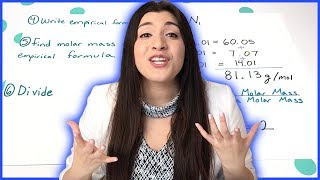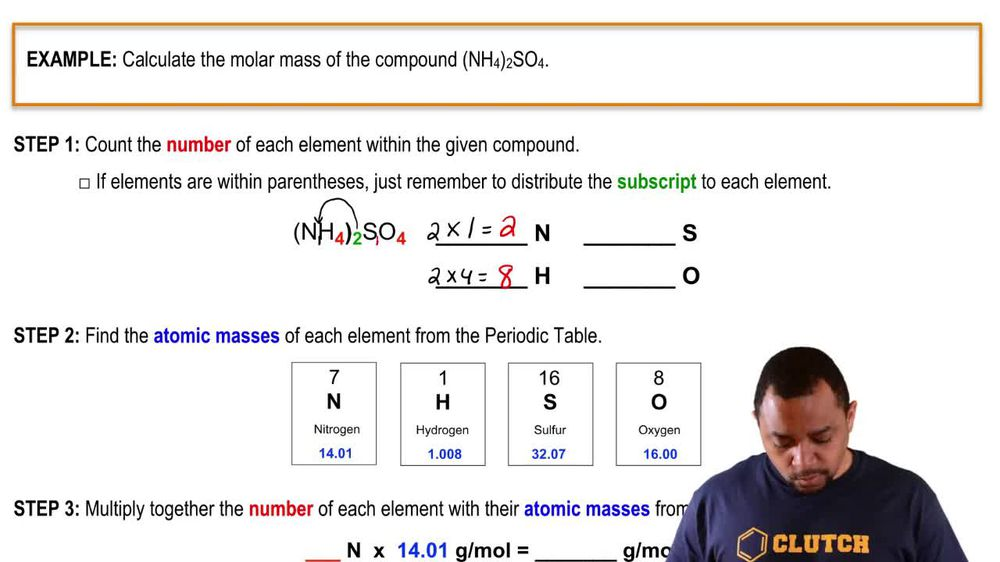Start typing, then use the up and down arrows to select an option from the list.
1. 2. Atoms & Elements2. Calculating Molar Mass
Problem

# Match the descriptions (a)–(e) with the following terms: atomic weight, atomic mass, mass number, atomic number, molar mass. (a) The mass of a specific atom such as one atom of 13C (b) The quantity determined by the number of protons in an element. (c) The number of grams in 1 mol of an element (d) The number of protons and neutrons in an element (e) The weighted average of the isotopic masses of an element’s naturally occurring isotopes

Relevant Solution1m
Play a video:
Hey everyone where asked what is the difference between atomic mass atomic weight and molar mass. So looking at our answer choices, let's go ahead and start with a for a, it tells us that the atomic mass is the sum of the number of protons and neutrons in an element. Now this is already wrong because the sum of the number of protons and neutrons in an element actually is our mass number. So that is not our atomic mass. Let's go ahead and look at B. So for B we have our atomic mass is the mass of a single atom of an element or isotope. This statement is correct. That is the definition of an atomic mass. For atomic weight we have the weighted average of the masses of an element's naturally occurring isotopes. This statement is also correct since that is the definition of atomic weight. And lastly looking at Mueller mass, it tells us that the mass in grams of one mole of an element or compound. And this statement is also correct. So it looks like B is going to be our final answer. But let's go ahead and look at C and D and determine why those are wrong. For C. Atomic mass has the correct definition. But for atomic weight, it tells us that this is the sum of the numbers of protons and neutrons in an element. And this is incorrect because again, that is our mass number for D we have our atomic mass tells us the number of protons in an element. This is also incorrect because the number of protons in an element tells us our atomic number. So I hope this made sense and let us know if you have any questions.